cancel
Showing results for
Did you mean:Post Prodigy

## Cumulative Total

Hi,

I'm at an absolute loss as to how to calculate a cumulative total. I've tried googling, reading the forums, following the documentation, decomposing the calculation, trying it as both a measure and a calc'd column; It always seems to refer me to the same number. So for example; March will be 10, April 12, but instead of showing me 10 for March and 22 for April, it shows me 10 for March and 12 for april.

For eg: https://gyazo.com/41bd333cedac290e6980772906ff0034 with a measure

I my Month column as a date column, I've tried using all kinds of features including, calc, sum, sumx, time based functions.

The commonly reccomended filter of [Date] <= MAX [Date] always returns an error. The Earlier function returns errors with concerns there isn't a function above it. Any help would be greatly appreciated; I've spent hours today trying to work this out and I get the feeling there is a difference in Pivottable dax and powerbi dax. I want to be able to shape and transform my data in powerbi, using dax in powerbi.

Any help would be greatly appreciated.

1 ACCEPTED SOLUTIONCommunity Champion

@ElliotP Sorry about the original post. It was from my phone and had typosOkay here is the formula for Running Total as a Calculated Column (prorerly formatted)

```Running Total COLUMN =
CALCULATE (
SUM ( 'All Web Site Data (2)'[UniquePageviews] ),
ALL ( 'All Web Site Data (2)' ),
'All Web Site Data (2)'[Date] <= EARLIER ( 'All Web Site Data (2)'[Date] )
)```

And as you can see it works!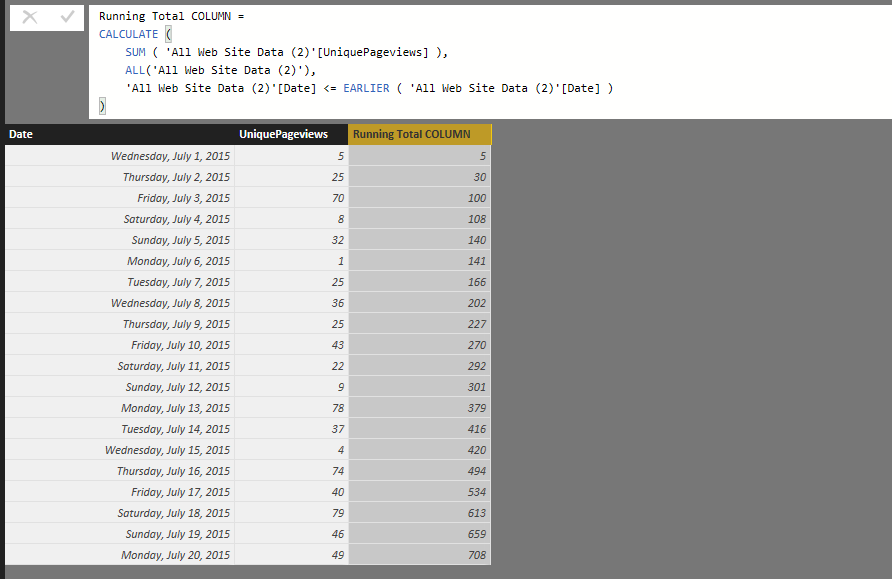And here's the MEASURE formula

```Running Total MEASURE =
CALCULATE (
SUM ( 'All Web Site Data (2)'[UniquePageviews] ),
FILTER (
ALL ( 'All Web Site Data (2)' ),
'All Web Site Data (2)'[Date] <= MAX ( 'All Web Site Data (2)'[Date] )
)
)```

Which also works...77 REPLIES 77Frequent Visitor

I FINALLY FIGURED OUT HOW TO DO THIS FOR A COLUMN OF STRINGS

There's definitely a better way but I started by making a calculated column which converts my strings to numbers. In the example below, I was counting all parts that were not "good"

Calculated Column:

converter column = IF('table'[quality] == "Good", 0, 1)

Running Total =
CALCULATE (
SUM ('table'[converter column] ),
ALLEXCEPT ('table', table[converter column]),
'table'[Tool Time] <= EARLIER ( 'table'[Tool Time] )
)Anonymous
Not applicable

Hello All,

I've tried several methods but unfortunately without luck 😞

I've an table with following columns:

• issue/ defect reported date
• Defect ID
• Repaired (Y/N)
• defect closure dateI would really appreciate, if anyone can help me on this.

Regards,

JaiAnonymous
Not applicable

Hi Jai,

Can I suggest you try using the TOTALYTD function?

As you can see in my example below, it's quite straight forward for Sum functions so it should be quite straight forward for your count functions too. It also gives you the ability to set the year end date so that it groups the correct months together. That is the "31/3" in my example string below:

Order Intake Margin YTD Current Month = TOTALYTD([Total Order Intake Margin],CALENDAR[Report Date],"31/3")Anonymous
Not applicable

Thanks a lot, finally it worked 😀

I've another measure to created with running total. For this, I've following columns:

• Issue repair status (Y/N)
• Issue closed date (available only if above column is "Y" otherwise blank)

And for this, I would like to create a measure, to show the cumulative based on the month (i.e. how many issues has been closed on which month)

Regards,

JaiCUMULATIVE SUM, date sensitive, solved at least for me:

BEWARE, all solutions provided before do not work if the dataset goes more in the past compared to the starting date you want to see in the Cumulative sum. Meaning, the solutions provided so far do not work if i want a Cumulative sum for Year-to-date 2022, if i have data for 2021, 2020... And i want the cumulative sum to adjust based on the year.

HERE A SOLUTION THAT WORKED FOR ME:

See the outcome pics below, it works even when changing year, only starting the Cumulative Sum from the beginning of the selected year!

The measure is essentially a conditional calculation between the first day of the selected year and the last day of the current period (e.g. month):

Nb of ship-to Cumulative Total =
IF([Nb of ship-to]<>0,
CALCULATE([Nb of ship-to],
DATESBETWEEN('Calendar'[Date], DATE(YEAR(MIN('Calendar'[Date])),1,1), 'Calendar'[LastDate])), "")

The IF function is only there to make sure i see blank cells in the months Year-to-go.

The LastDate measure is calculated as:

LastDate = Lastdate('Calendar'[Date])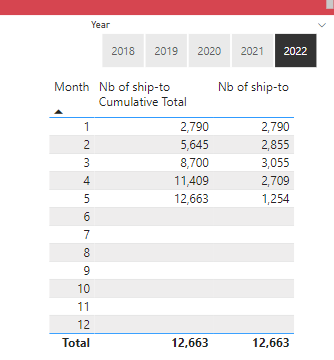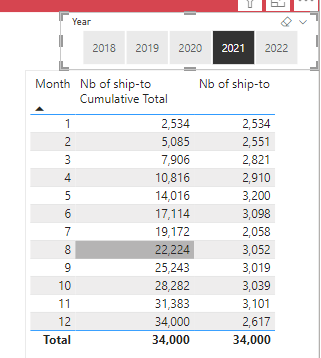Hello!

Something similar happens to me, I am looking for a function that shows me the accumulated per month for 2023 taking into account the values of 2020, 2021 and 2022, as follows:But I do not manage to make January start with the accumulated values of previous years and that from there it accumulates

Will you know how?

By the way, I found the function of "IF([]),<>0, "") very useful. Thank you!!Anonymous
Not applicable

Hi,

wouuld you like to solve my issue . I triying to get the cumulative flag according to %age.

one person have 4 channel if there 3 chanel fill 80% then previous three should be come in flag like "top 80%" and 4th should be "rest")

Hi its my humble request please solve this queryAnonymous
Not applicable

I Need help

for my question: IF my contri will completed 80% till any point then we have to make the 80% for each and every before meeted point : example given as " NEEd Ans like"Anonymous
Not applicable

Hi Legends!

Can I get some help with this please? As far as I can tell I've done exactly the right things to copy paste the solution but I can't get the correct result. Thanks in advance.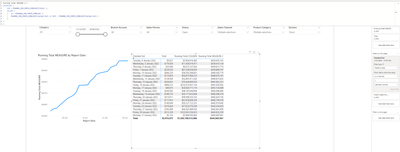Regular Visitor

Hi All,

Thanks for the previous answers to the initial poster. However, after reading all the posts on the subject i cannot find an answer to the following request:

I need to calculate a running total for QuantityBatches by Plan_Type, Product_ID and Calendar_date in order to make some stock projection and all of this sorted by the Calendar_Date.

Colonne =

calculate(

sum(View_Latest_Schedule[QuantityBatches]),

filter(

ALL(View_Latest_Schedule[Plan_Type]),

View_Latest_Schedule[Plan_Type] = "Frac_Plan"

)

View_Latest_Schedule[CalendarDate] <= EARLIER(View_Latest_Schedule[CalendarDate] )

)Anonymous
Not applicable

Hi There,

I tried as suggested but only get it working almost.Because there are no actuals from 01/01/2021 onwards the last value will be shown. How can I show the cumulative only if there is an actual value?

Actuals Cumulative =
CALCULATE(
SUM('Contract Revenue Forecast'[Actual]),
FILTER(
ALL('Contract Revenue Forecast'),
'Contract Revenue Forecast'[Month] <= EARLIER( 'Contract Revenue Forecast'[Month])
))

Cheers,Helper I

I used the solutions provided although I needed to reset the sum every week, this worked well for me. Added a variable for the week number to compare against. Adds each day as it goes until it reaches a new week, starts again.

``````Running SOP =
var x = SOP[Week Number]

return
CALCULATE (
SUM ( 'SOP'[Production Tonnes])
, ALL (SOP),
('SOP'[Date] <= EARLIER ( 'SOP'[Date]) && weeknum(SOP[Date],2) == x))``````New Member

Anyone know how to get cumulative total by site?

 Date_Time Site number 1/01/2015 6:00 AA 35 1/01/2015 6:00 BB 22 1/01/2015 6:00 CC 47 1/01/2015 18:00 AA 12 1/01/2015 18:00 BB 65 1/01/2015 18:00 CC 24 2/01/2015 6:00 AA 35 2/01/2015 6:00 BB 78 2/01/2015 6:00 CC 65 2/01/2015 18:00 AA 12 2/01/2015 18:00 BB 45 2/01/2015 18:00 CC 68 3/01/2015 6:00 AA 66 3/01/2015 6:00 BB 74 3/01/2015 6:00 CC 32 3/01/2015 18:00 AA 36 3/01/2015 18:00 BB 54 3/01/2015 18:00 CC 69 4/01/2015 6:00 AA 24 4/01/2015 6:00 BB 56 4/01/2015 6:00 CC 35 4/01/2015 18:00 AA 45 4/01/2015 18:00 BB 39 4/01/2015 18:00 CC 57 5/01/2015 6:00 AA 78 5/01/2015 6:00 BB 35 5/01/2015 6:00 CC 15Helper I

@drnareshchauhan

It's a bit hacky although you could create references of the table and filter into each site (If there were not too many) Use the solution supplied to accumulative sum based on the date. Then union all of the tables... although I am sure there must be a better way...
EDIT:
I am ashamed I even thought of that, a much better way to sum on two columns in your situation is using Python

``````# 'dataset' holds the input data for this script
dataset['cumsum'] = dataset.groupby(['site','datepd'])['number'].cumsum()``````

Notes:

Pandas does not like returning dates properly, create a duplicate column which is date type datepd of your date column.
Change your original date to text so you have a reference of the date afterwards.
Use the code above.

Select the dataset.

Remove the datepd column

Change the date column to date type again.

SHould be good to go.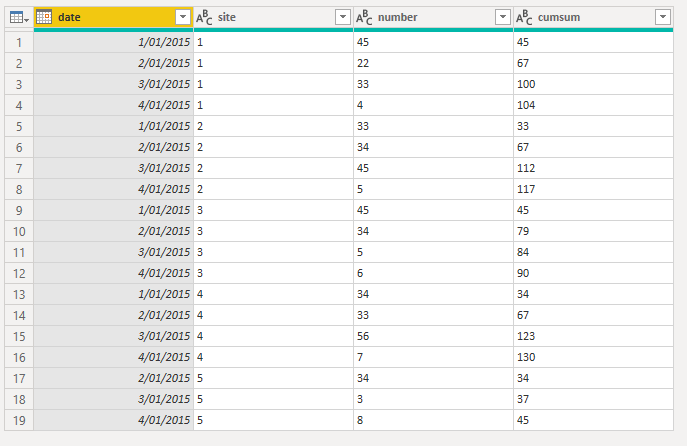Microsoft

Thanks for solution. Could you please suggest how we can wite DAX / Measure formula to get cumulative sum like this..Frequent Visitor

Why in measure we use MAX () but use Earlier () in the calculated column?  Are they exchangable ?New Member

Hi,

I have been trying to cumulative sum for a long time, but I couldnt it. I give the example below, İf you can help me I realy will be grateful. Thank you for your considiration. God bless you.

I try to 2017-01 + 2017-02 and try to write new sheet . I give you excel example.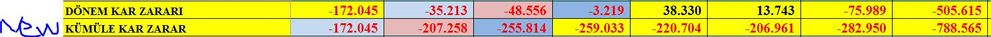Regular Visitor

Hi,

I am facing problems with getting the right DAX expressions.

The Running Total column is calculated manually... this is the result that I want to achieve with the Running Total Measure. The Running Total sums up for each Team and across the dates.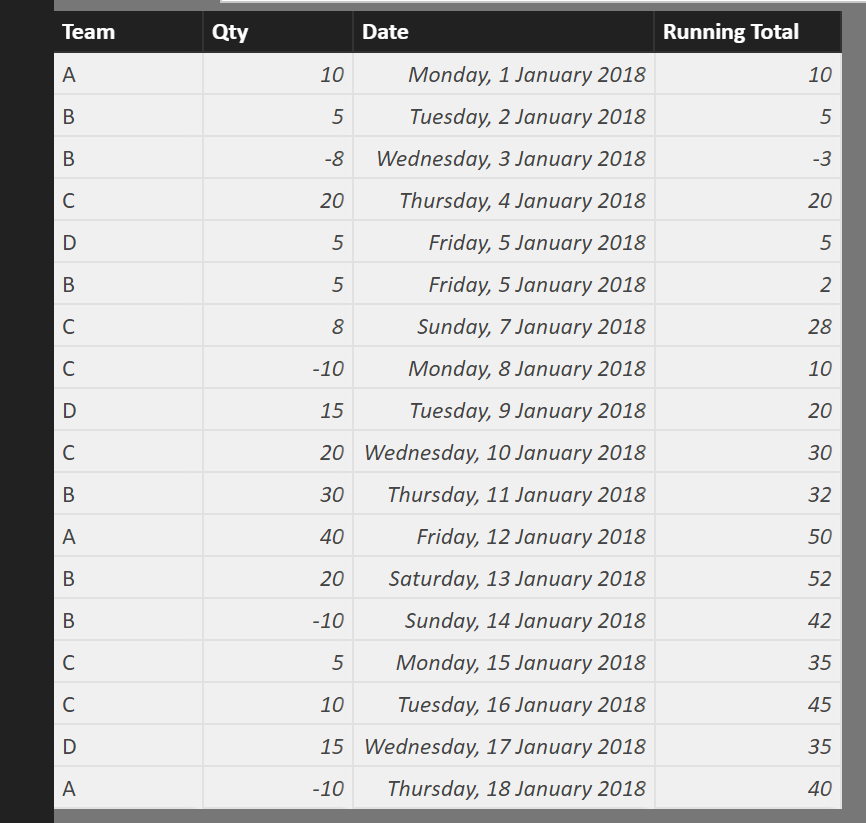I'm using the current DAX expression, but it is running total regardless of the Team.

Running Total Measure =
CALCULATE(
SUM(Sheet1[Qty]),FILTER(ALL(Sheet1),Sheet1[Date]<=MAX(Sheet1[Date])))

Can someone help? Thanks.Frequent Visitor

Hi,

I got this expression working in a bar chart so that it shows running total correctly when [Date] field is selected as x-axis. However, if I use date hierarchy as x-axis, it won't work anymore. Instead of running total, it gives period's total. E.g. if Total Sales in January is 100 and 200 in February, it displays 100 for January and 200 for February, instead of 100 for Jan and 300 for Feb as it should. Does anyone know what might be the issue?

```CALCULATE (
SUM ( [TotalSales] );
FILTER(
ALL ( [Sales] );
Sales[Date] <= MAX( Sales[Date] )
)
)```New Member

As I try to learn Power BI,

I have face the same question also but the data i have may have slightly different than the above situation.

I have a sample data which are the sales data of some countries.

I want to find the cumulative total sales but I have sales which happen more than once in the same day.

I have try to apply the above formula but its only apply on 'year' level but still the value are incorrect also.

Here is what I intend to get: Chart I want to have

Here are the sample data: Sample data picture

Here's the formula I try to apply: Formula of cumulative total I tried to apply

(where the date here are the 'Order Date' and with the value which is 'Sales')

Really appreciate if any one can have reply, if there is anything you want, I will provide.

Thank alot!